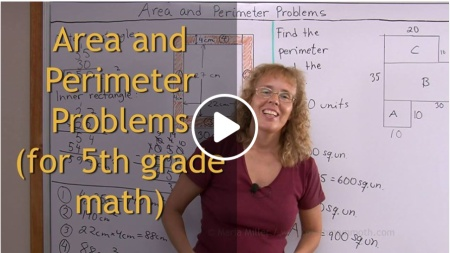^

# Free math video lessons for 5th gradeThis is a collection of free math videos for 5th grade, showing varied exercises for each topic. They match Math Mammoth Grade 5 curriculum but will also work no matter which curriculum you follow (in other words, the videos don't rely on you having Math Mammoth curriculum).

Please choose a topic from the list. I will expand the list as I'm able to edit and upload more videos.

## Chapter 1: The four operations

Bar models for addition and subtraction equations, plus addition and subtraction terms

Review of concepts related to multiplication and division: simple equations, bar models, terminology

Long division with a two-digit divisor

Long division as continued (repeated) subtraction

## Chapter 1: Number theory

Divisibility rules

(Introduction to primes — as a review from 4th grade)

Prime factorization using factor trees

## Chapter 2: Place value / large numbers

Powers of ten and exponents

Rounding large numbers

Example: finding powers of 7 with a calculator

Examples of calculator usage: word problems

## Chapter 3: Problem solving

Problem solving with bar models: fractional part of a whole

Using bar models: a word problem where total and difference are given

More problem solving with bar models, part 1

More problem solving with bar models, part 2

## Chapter 4: Decimals

Decimal place value and adding decimals, up to 2 decimal digits

Decimal place value (to thousandths)

Fractions to decimals, and comparing decimals — up to 3 decimal digits (thousandths)

Add & subtract decimals using mental math

Long division with decimals, plus fractions to decimals

## Chapter 5: Graphing and Statistics

Numerical patterns in the coordinate grid

Drawing a histogram and a double bar graph

Mean and mode

## Chapter 6: Decimals

Multiplying decimals by decimals: the shortcut

Multiplying decimals and whole numbers: scaling

Divide decimals with mental math, part 1: sharing divisions (whole-number divisor)

Divide decimals with mental math, part 2: divisor is a decimal

Multiply and divide decimals by powers of ten

Divide decimals by decimals

Decimal division: problem solving

Problem solving with decimals

Decimals in measuring units — introduction

Basics of the metric system and the prefixes

Rounding and estimating with measurements

## Chapter 7: Fractions: Add and Subtract

Mixed numbers to fractions and vice versa

Add mixed numbers (like fractional parts)

Subtract mixed numbers (like fractional parts)

Equivalent fractions

The least common denominator

Comparing fractions

## Chapter 8: Fractions: Multiply and Divide

Simplifying fractions

Multiply fractions by whole numbers

Multiply fractions by fractions

Fraction multiplication and area

Simplifying before multiplying

Multiplying mixed numbers

Multiplication as scaling/resizing

Fractions are divisions

Reciprocal numbers and the shortcut for fraction division

Divide fractions: mental math

How to divide fractions & reciprocal numbers

Ratios and fractions

## Chapter 9: Geometry

Draw a square with a given side, and copy a triangle

Draw a hexagon and measure its angles

Area and perimeter problems

Classify triangles

Volume of a rectangular prism and cubic units

WAIT!

Receive my monthly collection of math tips & resources directly in your inbox — and get a FREE Math Mammoth book!You can unsubscribe at any time.

### Math Mammoth Tour

Confused about the different options? Take a virtual email tour around Math Mammoth! You'll receive:

An initial email to download your GIFT of over 400 free worksheets and sample pages from my books. Six other "TOURSTOP" emails that explain the important things and commonly asked questions concerning Math Mammoth curriculum. (Find out the differences between all these different-colored series!)

This way, you'll have time to digest the information over one or two weeks, plus an opportunity to ask me personally about the curriculum.
A monthly collection of math teaching tips & Math Mammoth updates (unsubscribe any time)### "Mini" Math Teaching Course

This is a little "virtual" 2-week course, where you will receive emails on important topics on teaching math, including:

- How to help a student who is behind
- Troubles with word problems
- Teaching multiplication tables
- Why fractions are so difficult
- The value of mistakes
- Should you use timed tests
- And more!

A GIFT of over 400 free worksheets and sample pages from my books right in the very beginning.A monthly collection of math teaching tips & Math Mammoth updates (unsubscribe any time)
Enter your email to receive math teaching tips, resources, Math Mammoth news & sales, humor, and more! I tend to send out these tips about once monthly, near the beginning of the month, but occasionally you may hear from me twice per month (and sometimes less often).• A GIFT of over 400 free worksheets and sample pages from my books.